Courses

# Chapter 4 Fluid Kinematics - Fluid Mechanics, Mechanical Engineering Mechanical Engineering Notes | EduRev

## Mechanical Engineering : Chapter 4 Fluid Kinematics - Fluid Mechanics, Mechanical Engineering Mechanical Engineering Notes | EduRev

The document Chapter 4 Fluid Kinematics - Fluid Mechanics, Mechanical Engineering Mechanical Engineering Notes | EduRev is a part of the Mechanical Engineering Course Mechanical Engineering SSC JE (Technical).
All you need of Mechanical Engineering at this link: Mechanical Engineering

FLUID KINEMATICS

If the fluid and flow characteristics (such as density, velocity,  pressure etc.) at a point do not change with time, the flow is said to be steady flow. If the fluid and flow variables at a point may change with time, the flow will be unsteady. 
• Uniform and Non-uniform flow
If the velocity vector at all points in the flow is same at any instant of time, the flow is uniform flow. If the velocity vector varies from point to point at any instant of time, the flow will be non-uniform laminar flow generally occess at low velocities while turbulent flow occurs at high velocities. 
• Laminar and Turbulent flow
In laminar flow, the particles moves in layers sliding smoothly over the adjacent layers while in turbulent flow particles have the random and erratic movement, intermixing in the adjacent layers. 
• Streamline 
• A streamline is an imaginary line drawn in a flow field such that a tangent drawn at any point on this time represents the direction of velocity vector at that point. 
• There is no velocity component normal to stream lines.
• Pathline

A pathline is a curve traced by a single fluid particle during its motion. The path can cross each other. 

• Streakline
• When a dye is injected in a liquid or smoke in a gas so as to trace the subsequent motion of liquid particles passing a fixed point, the path followed by the dye or smoke is called the streakline. 
• In a steady flow streamline, pathline & streak lines are same. 
• Stream tube

Stream lines are drawn through a closed curve, they form a boundary surface across which fluid cannot penetrate. 

• Stream-line equation in 3- D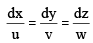Fluid Acceleration

Physically acceleration of any object is a measure of the change in its velocity. If the velocity vector of any fluid flow is a function of space and time, then it can change with space and as well as with time. Thus the acceleration, or change in velocity, experienced by the fluid particles can be due to the change of velocity with space and can be due to the change of velocity with time.

The acceleration of fluid particles due to change in velocity in space is called convective acceleration and acceleration due to change in velocity in time is called local or temporal acceleration. Acceleration of fluid particles can thus have two components: tangential and normal acceleration.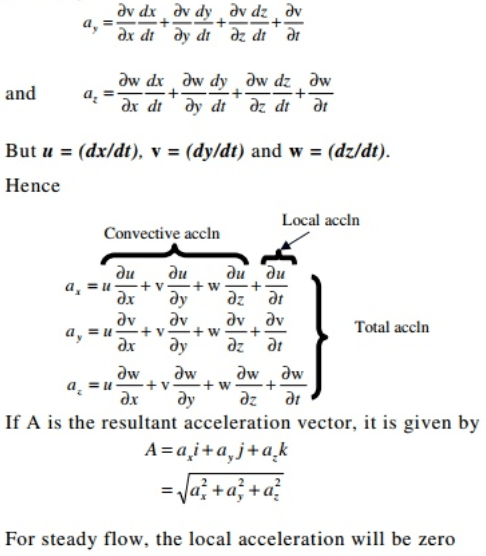• Tangential acceleration

Tangential acceleration is due to the change in velocity along the direction of motion. This tangential change in velocity or the tangential acceleration of fluid particles is the sum of tangential convective (change with space) and tangential local (change with time) accelerations.

• Normal acceleration

Normal acceleration of any particle is the component of the change in velocity normal to the direction of motion or the tangential velocity. Normal acceleration comes into picture when fluid particles move in curved paths. While moving in curved paths the velocity of the fluid particle changes in direction; it can also change in magnitude, too.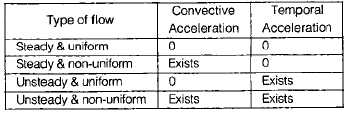• Continuity Equations
For incompressible and steady 3 dimensional flow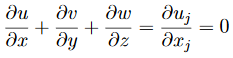For compressible and 3 dimensional unsteady flow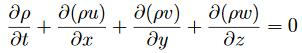For compressible one dimensional flow

r1 A1 V1 = r2 A2 V2

One dimensional differential form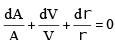For incompressible one dimensional flow

A1V1 = A2V2

Circulation

The circulation, C, about a closed contour in a fluid is defined as the line integral evaluated along the contour of the component of the velocity vector that is locally tangent to the contour.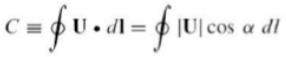Vorticity

In continuum mechanics, the vorticity is a pseudovector field that describes the local spinning motion of a continuum near some point (the tendency of something to rotate, as would be seen by an observer located at that point and traveling along with the flow.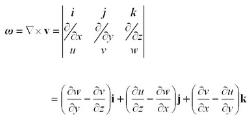The vorticity equation of fluid dynamics describes evolution of the vorticity ω of a particle of a fluid as it moves with its flow, that is, the local rotation of the fluid (in terms of vector calculus this is the curl of the flow velocity). The equation is:

D                                              ω                                                                                    D                      t                                                                                                                  =                                                                            ∂                                              ω                                                                                    ∂                      t                                                                      +                (                                  u                                ⋅                ∇                )                                  ω                                                                                                                  =                (                                  ω                                ⋅                ∇                )                                  u                                −                                  ω                                (                ∇                ⋅                                  u                                )                +                                                      1                                          ρ                                              2                                                                                            ∇                ρ                ×                ∇                p                +                ∇                ×                                  (                                                                                    ∇                        ⋅                        τ                                            ρ                                                        )                                +                ∇                ×                                  (                                                                                    B                                            ρ                                                        )                                                                          {\displaystyle {\begin{aligned}{\frac {D{\boldsymbol {\omega }}}{Dt}}&={\frac {\partial {\boldsymbol {\omega }}}{\partial t}}+(\mathbf {u} \cdot \nabla ){\boldsymbol {\omega }}\\&=({\boldsymbol {\omega }}\cdot \nabla )\mathbf {u} -{\boldsymbol {\omega }}(\nabla \cdot \mathbf {u} )+{\frac {1}{\rho ^{2}}}\nabla \rho \times \nabla p+\nabla \times \left({\frac {\nabla \cdot \tau }{\rho }}\right)+\nabla \times \left({\frac {\mathbf {B} }{\rho }}\right)\end{aligned}}}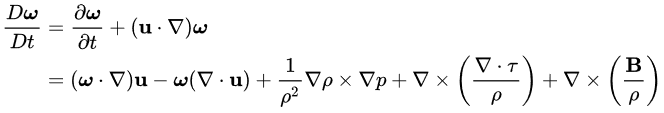where Dt is the material derivative operator, u is the flow velocity, ρ is the local fluid density, p is the local pressure, τ is the viscous stress tensor and B represents the sum of the external body forces.

Irrotational and Rotational flow 

• In irrotational flow, the vorticity is zero, at all points in the flow region while for rotational flow, vorticity is non–zero. 
• Flow outside the boundary layer has irrotational characteristic while that within the boundary layer is rotational characteristic. 

 Velocity potential (ϕ)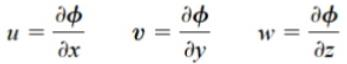• Flow always occurs in the direction of decreasing potential. 
• If f exists and satisfies continuity equation, then it represents the possible irrotational flow. 
• If f satisfies Laplace equation then it is the case of steady incompressible and irrotational flow. 
• Laplace equation is given by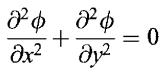 Streamline function ( y )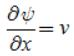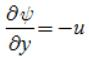• If y exists, then it satisfies continuity equation which can be rotational or irrotational. 
• If stream function satisfies Laplace equation then it is case of irrotational flow. 

Flow net

• A flownet is a graphical representation of two-dimensional steady-state groundwater flow through aquifers.

Construction of a flownet is often used for solving groundwater flow problems where the geometry makes analytical solutions impractical.

Offer running on EduRev: Apply code STAYHOME200 to get INR 200 off on our premium plan EduRev Infinity!

83 docs|53 tests

,

,

,

,

,

,

,

,

,

,

,

,

,

,

,

,

,

,

,

,

,

,

,

,

;